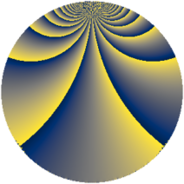# Properties

 Label 405.4.tLevel $405$ Weight $4$ Character orbit 405.t Rep. character $\chi_{405}(4,\cdot)$ Character field $\Q(\zeta_{54})$ Dimension $2880$ Sturm bound $216$

# Related objects

## Defining parameters

 Level: $$N$$ $$=$$ $$405 = 3^{4} \cdot 5$$ Weight: $$k$$ $$=$$ $$4$$ Character orbit: $$[\chi]$$ $$=$$ 405.t (of order $$54$$ and degree $$18$$) Character conductor: $$\operatorname{cond}(\chi)$$ $$=$$ $$405$$ Character field: $$\Q(\zeta_{54})$$ Sturm bound: $$216$$

## Dimensions

The following table gives the dimensions of various subspaces of $$M_{4}(405, [\chi])$$.

Total New Old
Modular forms 2952 2952 0
Cusp forms 2880 2880 0
Eisenstein series 72 72 0

## Trace form

 $$2880 q - 36 q^{4} - 18 q^{5} - 36 q^{6} - 36 q^{9} + O(q^{10})$$ $$2880 q - 36 q^{4} - 18 q^{5} - 36 q^{6} - 36 q^{9} - 18 q^{10} - 36 q^{11} - 36 q^{14} - 18 q^{15} - 36 q^{16} - 36 q^{19} + 558 q^{20} - 252 q^{21} - 36 q^{24} - 18 q^{25} + 2790 q^{26} - 36 q^{29} - 774 q^{30} - 36 q^{31} - 36 q^{34} + 1224 q^{35} - 4644 q^{36} - 36 q^{39} - 18 q^{40} + 1818 q^{41} - 2700 q^{44} - 1044 q^{45} - 36 q^{46} - 36 q^{49} + 4779 q^{50} - 5112 q^{51} + 6642 q^{54} - 9 q^{55} - 6102 q^{56} + 2448 q^{59} + 1269 q^{60} - 36 q^{61} - 36 q^{64} - 4284 q^{65} + 7380 q^{66} + 342 q^{69} - 18 q^{70} - 36 q^{71} - 5508 q^{74} - 18 q^{75} - 36 q^{76} + 4176 q^{79} - 36 q^{80} - 36 q^{81} - 17658 q^{84} - 18 q^{85} - 36 q^{86} - 1476 q^{89} - 12555 q^{90} - 36 q^{91} - 5544 q^{94} - 5310 q^{95} - 36 q^{96} + 9396 q^{99} + O(q^{100})$$

## Decomposition of $$S_{4}^{\mathrm{new}}(405, [\chi])$$ into newform subspaces

The newforms in this space have not yet been added to the LMFDB.